# Unimodal distribution

Jump to: navigation, search

single-peak distribution

A probability measure on the line whose distribution function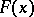is convex forand concave for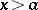for a certain real. The numberin this case is called the mode (peak) and is, generally speaking, not uniquely determined; more precisely, the set of modes of a given unimodal distribution forms a closed interval, possibly degenerate.

Examples of unimodal distributions include the normal distribution, the uniform distribution, the Cauchy distribution, the Student distribution, and the "chi-squared" distribution. A.Ya. Khinchin  has obtained the following unimodality criterion: For a functionto be the characteristic function of a unimodal distribution with mode at zero it is necessary and sufficient that it admits a representation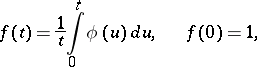whereis a characteristic function. In terms of distribution functions this equation is equivalent to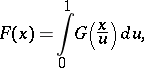whereandcorrespond toand. In other words,is unimodal with mode at zero if and only if it is the distribution function of the product of two independent random variables one of which has a uniform distribution on.

For a distribution given by its characteristic function (as e.g. for a stable distribution) the proof of its unimodality presents a difficult analytical problem. The seemingly natural way of representing a given distribution as a limit of unimodal distributions does not achieve this aim, because in general the convolution of two unimodal distributions is not a unimodal distribution (although for symmetric distributions unimodality is preserved under convolution; for a long time it was assumed that this would be so in general). For example, ifis the probability distribution with an atom of size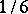at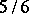and a densitythen the density of the convolution ofwith itself has two maxima. Therefore the concept of strong unimodality has been introduced (cf. ); a distribution is said to be strongly unimodal if its convolution with any unimodal distribution is unimodal. Every strongly unimodal distribution is unimodal.

A lattice distribution giving probability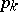to the point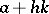,, is called unimodal if there exists an integer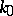such that, as a function of, is non-decreasing forand non-increasing for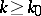. Examples of unimodal lattice distributions are the Poisson distribution, the binomial distribution and the geometric distribution.

Certain results concerning distributions may be strengthened by assuming unimodality. E.g. the Chebyshev inequality in probability theory for a random variablehaving a unimodal distribution may be sharpened as follows: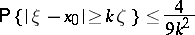for any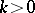, whereis the mode and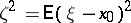.